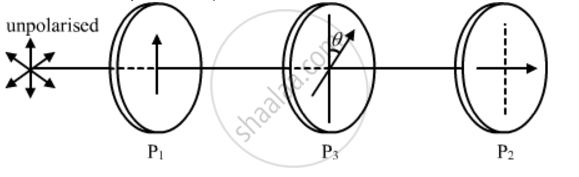Share

Two Polaroids P1 and P2 Are Placed with Their Pass Axes Perpendicular to Each Other. an Unpolarised Light of Intensity Io is Incident on P1. - CBSE (Science) Class 12 - Physics

Question

Two polaroids P1 and P2 are placed with their pass axes perpendicular to each other. An unpolarised light of intensity Io is incident on P1. A third polaroid P3 is kept in between P1 and P2 such that its pass axis makes an angle of 45° with that of P1. Determine the intensity of light transmitted through P1, P2 and P3

Solution

As given in the question, the polaroids P1 and P2 are placed with their pass axes perpendicular to each other. Also, P3 is placed at an angle of 45° with respect to P1.Now, we have:

Intensity of light after falling on PI'=I_0/2

Intensity of light after falling on P3 I"=

I'cos^2(theta)=I_0/2cos^2(45^@)=I_0/4

Therefore, a light of intensity I_0/4will pass through P3 and the angle between P3 and P2 will be 45° because of the condition given in the question.

Intensity of light after falling on PI''' = I'cos^2(theta)=I_0/4cos^2(45^@)=I_0/8

Is there an error in this question or solution?

Video TutorialsVIEW ALL 

Solution Two Polaroids P1 and P2 Are Placed with Their Pass Axes Perpendicular to Each Other. an Unpolarised Light of Intensity Io is Incident on P1. Concept: Polarisation.
S